Home
Hostname: page-component-568f69f84b-jtg5s Total loading time: 0.157 Render date: 2021-09-18T06:04:38.281Z Has data issue: true Feature Flags: { "shouldUseShareProductTool": true, "shouldUseHypothesis": true, "isUnsiloEnabled": true, "metricsAbstractViews": false, "figures": true, "newCiteModal": false, "newCitedByModal": true, "newEcommerce": true, "newUsageEvents": true }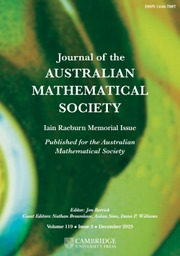Journal of the Australian Mathematical Society

## Abstract

Let$g$ be an element of a finite group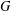$G$ and let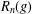$R_{n}(g)$ be the subgroup generated by all the right Engel values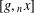$[g,_{n}x]$ over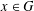$x\in G$ . In the case when$G$ is soluble we prove that if, for some$n$ , the Fitting height of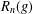$R_{n}(g)$ is equal to$k$ , then$g$ belongs to the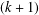$(k+1)$ th Fitting subgroup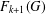$F_{k+1}(G)$ . For nonsoluble$G$ , it is proved that if, for some$n$ , the generalized Fitting height of$R_{n}(g)$ is equal to$k$ , then$g$ belongs to the generalized Fitting subgroup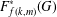$F_{f(k,m)}^{\ast }(G)$ with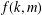$f(k,m)$ depending only on$k$ and$m$ , where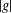$|g|$ is the product of$m$ primes counting multiplicities. It is also proved that if, for some$n$ , the nonsoluble length of$R_{n}(g)$ is equal to$k$ , then$g$ belongs to a normal subgroup whose nonsoluble length is bounded in terms of$k$ and$m$ . Earlier, similar generalizations of Baer’s theorem (which states that an Engel element of a finite group belongs to the Fitting subgroup) were obtained by the first two authors in terms of left Engel-type subgroups.

## MSC classification

Type
Research Article
Information
Journal of the Australian Mathematical Society , December 2020 , pp. 340 - 350
© 2019 Australian Mathematical Publishing Association Inc.

## Access options

Get access to the full version of this content by using one of the access options below. (Log in options will check for institutional or personal access. Content may require purchase if you do not have access.)

## Footnotes

Communicated by B. Martin

The first author was supported by the Russian Science Foundation, project no. 14-21-00065, the second by FAPDF, Brazil, and the third by EPSRC.

## References

Baer, R., ‘Engelsche Elemente Noetherscher Gruppen’, Math. Ann. 133 (1957), 256270.Google Scholar
Hall, P. and Higman, G., ‘On the p-length of p-soluble groups and reduction theorems for Burnside’s problem’, Proc. Lond. Math. Soc. (3) 6 (1956), 142.Google Scholar
Khukhro, E. I. and Shumyatsky, P., ‘Words and pronilpotent subgroups in profinite groups’, J. Aust. Math. Soc. 97 (2014), 343364.Google Scholar
Khukhro, E. I. and Shumyatsky, P., ‘Engel-type subgroups and length parameters of finite groups’, Israel J. Math. 222 (2017), 599629.Google Scholar
Wilson, J. S., ‘On the structure of compact torsion groups’, Monatsh. Math. 96 (1983), 5766.Google Scholar
1
Cited by

# Send article to Kindle

Note you can select to send to either the @free.kindle.com or @kindle.com variations. ‘@free.kindle.com’ emails are free but can only be sent to your device when it is connected to wi-fi. ‘@kindle.com’ emails can be delivered even when you are not connected to wi-fi, but note that service fees apply.

Find out more about the Kindle Personal Document Service.

RIGHT ENGEL-TYPE SUBGROUPS AND LENGTH PARAMETERS OF FINITE GROUPS
Available formats
×

# Send article to Dropbox

To send this article to your Dropbox account, please select one or more formats and confirm that you agree to abide by our usage policies. If this is the first time you use this feature, you will be asked to authorise Cambridge Core to connect with your <service> account. Find out more about sending content to Dropbox.

RIGHT ENGEL-TYPE SUBGROUPS AND LENGTH PARAMETERS OF FINITE GROUPS
Available formats
×

# Send article to Google Drive

To send this article to your Google Drive account, please select one or more formats and confirm that you agree to abide by our usage policies. If this is the first time you use this feature, you will be asked to authorise Cambridge Core to connect with your <service> account. Find out more about sending content to Google Drive.

RIGHT ENGEL-TYPE SUBGROUPS AND LENGTH PARAMETERS OF FINITE GROUPS
Available formats
×
×